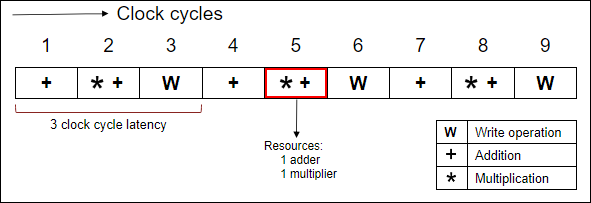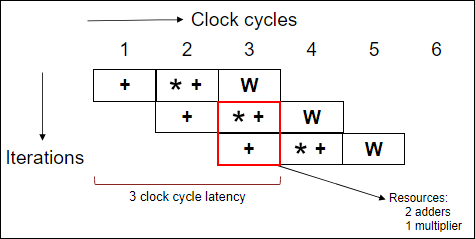## Pipelining of `for`-Loops

Pipelining allows concurrent execution of multiple iterations. The next iteration of a loop can begin execution before the previous iteration completes its execution. Pipelining optimises the execution speed and improves the throughput of the code at the expense of increased resources.

In MATLAB® you can pipeline `for`-loops by using `coder.hdl.loopspec(‘pipeline’) `or `coder.hdl.loopspec(‘pipeline’,initiation_interval)`.

To understand the concept of pipelining of `for`-loops, consider the following MATLAB code. It consists of a `for`-loop and a persistent array `arr`, mapped to RAM during code generation.

```for i = 1:20​ tmp = fi((a + b) * c + tmp, 0, 32, 0, hdlfimath);​ arr(i) = tmp;​ end​```

• Non-pipelined for-loop

In a non-pipelined loop, all the iterations of the `for`-loop are scheduled serially. The next iteration executes after the previous iteration completes its execution. Also, there is no overlap in the execution of the iterations in a non-pipelined loop. The following diagram shows the schedule of a non-pipelined `for`-loop.• Pipelined for-loop

In a pipelined loop, the next iteration start its execution with a gap of an initiation interval. This can lead to overlap in the execution of the iterations. The initiation interval represents the number of clock cycles before the start of the next iteration of the `for`-loop. The following diagram shows the schedule of a pipelined `for`-loop with an initiation interval of `1`.In the above schedules, a non-pipelined `for`-loop takes 9 clock cycles to complete 3 iterations whereas a pipelined `for`-loop takes only 5 clock cycles for 3 iterations. This shows the impact of pipelining in improving the throughput of the code at the expense of additional resources.

### Issues with Pipelined `for`-Loops

Pipelining enables resource sharing between multiple iterations, thus leading to the following hazards:

1. Conflicting Read and Write Operations

If a pipelined `for`-loop is performing read and write operations in the same iteration, it can lead to overlapping of these operations. The memory read operation in the next iteration can be scheduled before the memory write operation in the current iteration. This leads to reading of incorrect values between the `for`-loop iterations of the shared resources.

Consider the following MATLAB code and its testbench. The code consists of a pipelined `for`-loop with an `initiation_interval` of `1`, that performs both read and write operations in each iteration.

MATLAB CodeGenerated SystemC™ Code
```function out = f(in) persistent arr1; if isempty(arr1) arr1 = zeros(1,102); end coder.hdl.loopspec('pipeline',1); for i = 4:100 y = arr1(i-3); arr1(i) = in; end out = y; end ```
```% MATLAB Testbench a = fi(4,0,3,0); for i = 1:100 out(i) = f(a); end ```
```class f_fixptClass { public: sc_uint<3> f_fixpt_arr1; void f_fixpt_initialize_ram_vars() { int32_T t_0; L1: for (t_0 = 0; t_0 < 102; t_0 = t_0 + 1) { f_fixpt_arr1[t_0] = sc_uint<3>(0.0); } } sc_uint<3> f_fixpt(sc_uint<3> in) { sc_uint<3> out; L2: for (int32_T i = 0; i < 97; i = i + 1) { HLS_PIPELINE_LOOP( HARD_STALL, 1, "L2" ); out = f_fixpt_arr1[i]; f_fixpt_arr1[i + 3] = in; } return out; } }; ```

During synthesis, the high level synthesis (HLS) tool throws an error stating: ```Unable to guarantee the safety of the schedule in the pipelined loop```.

Solution:

To overcome the conflicting read and write operations, you can use any one of the following solution based on your design requirement.

• Use `coder.hdl.arraydistance(arr1,'max',1)`, which ensures that read and write operations enclosed in the `for`-loop are separated with a maximum array distance of one clock cycle.

```coder.hdl.loopspec('pipeline',1); for i = 4:100 coder.hdl.arraydistance(arr1,'max',1); y = arr1(i-3); arr1(i) = in; end```
• Alternatively, you can update the value of `initiation_interval` to `2` in the `coder.hdl.loopspec` pragma.

```coder.hdl.loopspec('pipeline',2); for i = 4:100 y = arr1(i-3); arr1(i) = in; end```

2. Limited Memory Access

In many designs, it is common to have a single loop performing two or more accesses to a single memory (RAM). If such a loop is pipelined with `initiation_interval` as 1, these memory accesses occur simultaneously during each clock cycle. Dual-port RAMs have two ports at maximum, so at most two parallel independent accesses can be scheduled to the memory in a single clock cycle.

However, with a pipelined `for`-loop, more than two memory accesses can occur in a single clock cycle. This scenario leads to a limited memory access issue.

Consider the following MATLAB code and its testbench. It has a persistent array `arr1` that is mapped to RAM. The `for`-loop is pipelined with an `initiation_interval` of `1`, and three write operations are performed on the RAM mapped variable `arr1` inside the `for`-loop body.

MATLAB CodeGenerated SystemC Code
```function out = f(in1, in2) persistent arr1; if isempty(arr1) arr1 = zeros(1,102); end coder.hdl.loopspec('pipeline',1); for i = 1:100 arr1(i) = in1+in2; arr1(i+1) = in1*2; arr1(i+2) = in1+in2-2; end out = sum(arr1); end ```
```% MATLAB Testbench a = int8(4); b = int8(5); out = f(a,b);```
```class f_fixptClass { public: sc_uint<4> f_fixpt_arr1; void f_fixpt_initialize_ram_vars() { int32_T t_0; L1: for (t_0 = 0; t_0 < 102; t_0 = t_0 + 1) { f_fixpt_arr1[t_0] = sc_uint<4>(0.0); } } sc_uint<10> f_fixpt(sc_uint<3> in1, sc_uint<3> in2) { sc_uint<10> out; sc_uint<11> Y; L2: for (int32_T i = 0; i < 100; i = i + 1) { HLS_PIPELINE_LOOP( HARD_STALL, 1, "L2" ); f_fixpt_arr1[i] = (sc_uint<4>)in1 + (sc_uint<4>)in2; f_fixpt_arr1[i + 1] = (sc_uint<4>)(in1 * sc_uint<2>(2.0)); f_fixpt_arr1[i + 2] = ((sc_uint<4>)in1 + (sc_uint<4>)in2) - (sc_uint<4>) sc_uint<5>(2.0); } Y = (sc_uint<11>)f_fixpt_arr1; L3: for (int32_T k = 0; k < 101; k = k + 1) { Y = (sc_uint<11>)((sc_uint<12>)Y + (sc_uint<12>)f_fixpt_arr1[k + 1]); } out = (sc_uint<10>)Y; return out; } };```

During synthesis, the HLS tool throws an error stating: ```Unable to produce a valid schedule, found 1 Violation(s)```.

Solution:

To overcome the limited memory access create a pipelined `for`-loop with an `initiation_interval` of `2`. This action ensures that two memory accesses occur in each iteration of the `for`-loop.

```coder.hdl.loopspec('pipeline',2); for i = 1:100 arr1(i) = in1+in2; arr1(i+1) = in1*2; arr1(i+2) = in1+in2-2; end```